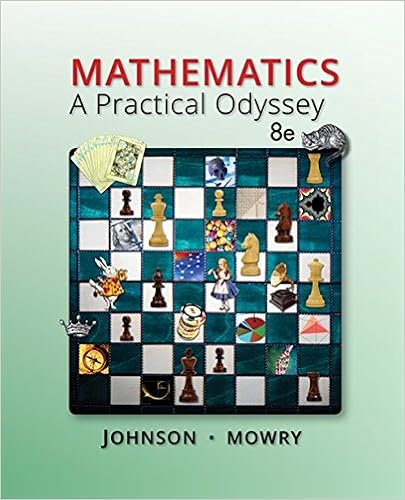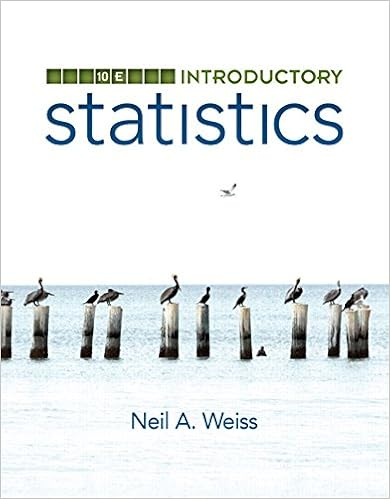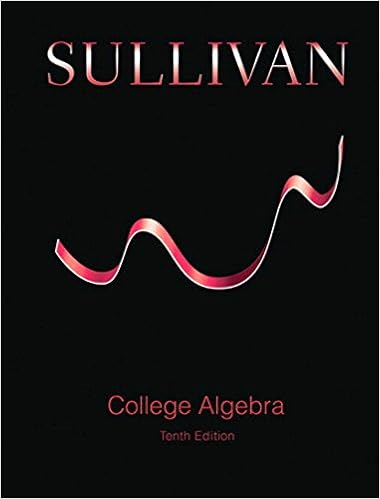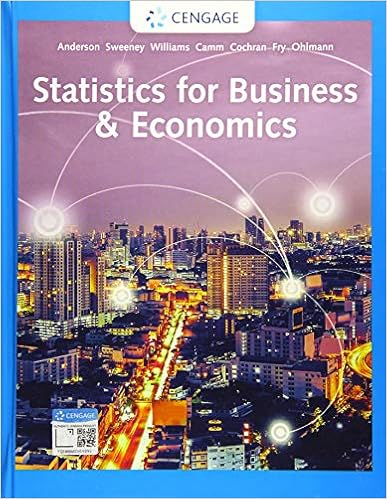# Practice Exam 2 on Finite Math - Math 1711 Finite Math...

• Test Prep
• 1

This preview shows page 1 out of 1 page.

Math 1711Finite MathSpring ’14Practice Exam 21.An experiment consists of picking a number at random from the set{1,2,3,4,5,6,7,8,9}.What is the probability that the number selected is 4? larger than 6?2.Are the following probabilities feasible for an experiment having sample space{s1, s2, s3}:Pr(s1) =.3,Pr(s2) =.5,Pr(s3) =.3?3.If the probability of a major earthquake in California this year is.65, then is ittrue that the odds against an earthquake are 35 to 65?4.An urn contains five white balls and four green balls.An experiment consistsof pulling 3 balls from the urn, one at a time without replacement.Find theprobability that all three balls selected are green. Find the probability that all 3balls are white if it is assumed that the last one selected is white.5.The 15 members of a senate committee will vote next week on an issue: 10 willvote “yes” and 5 will vote “no”. If a reporter samples 6 of the senators in order topredict the outcome of next week’s vote, what is the probability that the reportercorrectly predicts the outcome of the vote?
##### We have textbook solutions for you!The document you are viewing contains questions related to this textbook.
Chapter 3 / Exercise 6
Mathematics: A Practical Odyssey
Johnson/MowryExpert VerifiedBrowse all Textbook Solutions
End of preview. Want to read the entire page?

Course Hero member to access this document

Term
Fall
Professor
Evans
Tags
Math, Probability theory
##### We have textbook solutions for you!
The document you are viewing contains questions related to this textbook.The document you are viewing contains questions related to this textbook.
Chapter 3 / Exercise 6
Mathematics: A Practical Odyssey
Johnson/MowryExpert Verified
•••